# Other articles

1. Published: Mon 27 August 2018

# Haskell Countdown Solver

I love watching 8 Out Of 10 Cats Does Countdown. It's a british panel show where a few comedians solve word puzzles and mathematics puzzles. It's based on the game show Countdown that's been running since 1982.

And I also like the host Jimmy Carr very much.

## The Puzzles

The show consists of three different puzzles:

In the Letters round a team (or single contestant) picks 9 consonants and vowels from their respective piles. After the team chooses from one of both piles, the letter is revealed and put up on the board. They then choose again until 9 letters are on the board. After all letters are on the board, the teams have 30 seconds to form a word out of as many of the letters as possible. The team with the longest word scores that many points.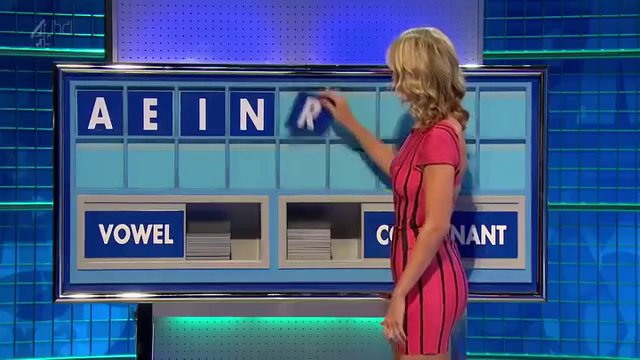Letters Round

In the Conundrum a list of letters that already spell out a funny group of words is given by the host and the contestants try to find a 9 letter anagram. The first team to buzzer in the correct answer gains 10 points. This is the final round of the game.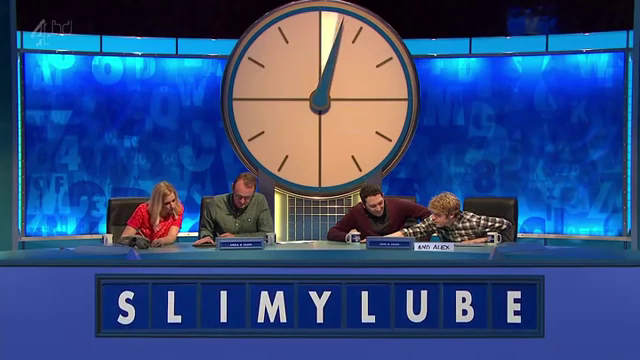Conundrum

In the Numbers round a team (or single contestant) picks 6 numbers from two piles. A random number is picked and the contestants have to create a mathematical formula out of the picked numbers that equal the target number. The specific rules follow.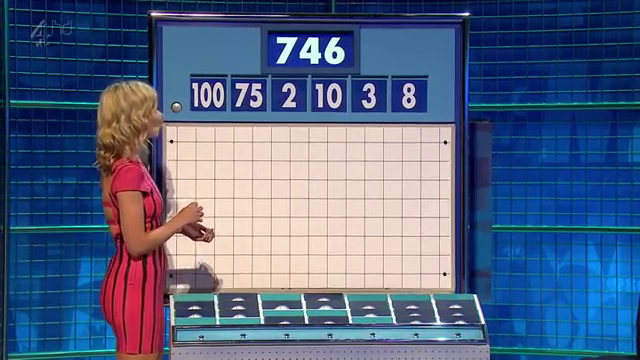Numbers Round

## Numbers Round

According to Wikipedia there are 6 columns of 4 numbers (4 rows). One column contains the numbers 25, 50, 75, and 100 and the other 5 columns contain the numbers 1 to 10 twice each. That's not exactly the same as on the show, as there appear to be 7 columns of 4 numbers but not every stack has all 4 number cards.

Contestants choose a total of 6 numbers from the "small" and "large" columns. The usual pick would be 2 to 3 "large" numbers and the rest small ones.

Then a random number between 100 und 999 is generated. The contestants try to create a mathematical formula that has the random number as the result. In the formulare only the following operations are allowed:

• Multiplication
• Subtraction (that does not result in a negative number)
• Division (that results in an integer)

The contestants do not have to use all the numbers to get the target number.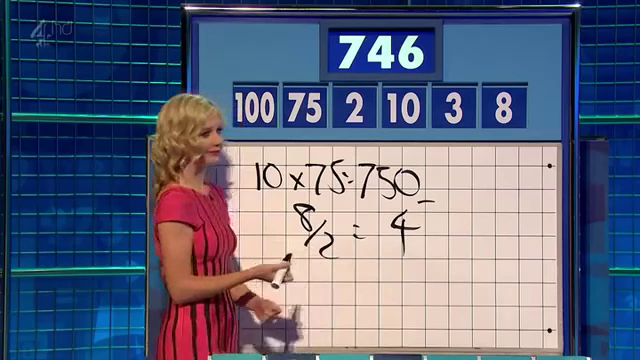Finished Numbers Round

Since I am really not good at this, I wrote a solver for the numbers game in Haskell.

## Solver

How do we go about this problem? First of all, we have to decide on a data structure. Let's start with a traditional term structure where a Term is a binary operation and two terms, or an integer value. An Operation is the function mapping two integers to a third, and a string to pretty-print the operation. The mapping is not total and might fail.

data Term = Op Operation Term Term | Val Integer
data Operation = Operation {fun :: (Integer -> Integer -> Maybe Integer), name :: String}

instance Show Term where
show (Val x) = show x
show (Op op l r) = "(" ++ (show l) ++ (name op) ++ show r ++ ")"


Now we have to define our operations and what they should do. Since we're using Maybe, and it's a monad, we can use monadic notation where it suits us:

operations = [  Operation (\x y -> return (x + y)) "+"
,  Operation (\x y -> return (x * y)) "*"
,  Operation (\x y -> guard (x > y) >> return (x - y)) "-"
,  Operation (\x y -> guard (x mod y == 0) >> return (x div y)) "/"
]
eval :: Term -> Maybe Integer
eval (Val v) = Just v
eval (Op o l r) = do l' <- eval l
r' <- eval r
(fun o) l' r'


As we can see, the preconditions to the subtraction and division are encoded using the guard function. If the function returns true, the whole operation returns Nothing, otherwise it returns Just the value.

Since Haskell uses lazy evaluation, we can define a list of all possible terms given a list of integers and filter for the ones that evaluate to the correct number.

As we create a Term we have to be careful to not use any of the numbers again. So we will split our numbers in two sets. One that can be used in the left Term of an Operation and the rest can be used in the right Term. We will create all terms using our definition. A Term is either the Value of one of the numbers for that subterm, or an operation with some numbers reserved for the left term and some numbers reserved for the right term.

terms :: [Integer] -> [Term]
terms nums =  do  n <- nums -- choose a number
[Val n] -- one solution
++
do  op <- operations -- choose an operation
(l, r) <- subset_split nums -- choose a split
guard $l /= [] guard$ r /= []
ls <- (terms l) -- choose a term for the left side
guard (eval ls /= Nothing)
rs <- (terms r) -- choose a term for the right side
guard (eval rs /= Nothing)
[Op op ls rs] -- one solution


For performance reasons we already evaluate the generated subterms in order to check whether the operations are valid. There are a lot of terms that can not be evaluated and we want to throw them out as early as possible (or not even generate them).

We haven't defined subset_split yet. The idea is, to just split the list of numbers in two groups in every possible way:

subsets :: [Integer] -> [[Integer]]
subsets []  = [[]]
subsets (x:xs) = subsets xs ++ map (x:) (subsets xs)

subset_split nums = do l <- subsets nums
return (l, nums \\ l)


Now all that remains is to generate all terms given a set of numbers, evaluate them, filter out the ones that don't have the correct value, and return the first one:

solve :: [Integer] -> Integer -> Maybe Term
solve nums target = let possible_solutions = map (\x -> (x, eval x)) (terms nums)
solutions = filter (\x -> (snd x) == (Just target)) possible_solutions
in listToMaybe $map fst solutions  And in our main we only read the list of numbers and the target as arguments and print the solution: main = do args <- getArgs let nums = (read (args !! 0))::[Integer] target = (read (args !! 1))::Integer print$ solve nums target


And there we go:

$./countdown "[100, 75, 2, 10, 3, 8]" 746 Just (8+(3+((75*(100-2))/10)))  Our solution is $$8+3+{{75*(100-2)} \over 10}$$ while the solution from the show was $$10*75 - {8 \over 2}$$. As you can see, we do not always get the easiest solution but we usually have a solution quite fast. This one took about a tenth of a second. The 30 seconds given to the contestant are usually quite enough. To make sure we had the "nicest" solution, we probably would need to generate all terms and sort them by size. The smallest one should be the nicest one. 2. Published: Mon 21 March 2016 # Solving Tatham's Puzzles - Signpost (backtracking) I've started writing solvers for games of Simon Tatham's Portable Puzzle Collection. Those Problems can usually be reduced to some NP-complete problem. This is quite a fun exercise.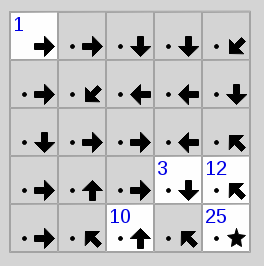Unsolved Signpost Puzzle We will be continuing with the puzzle "Signpost", which is a variant of Hamiltonian paths in a directed graph where, for some fields or nodes, the position within the path is given. The goal of the puzzle is to connect the Node "1" to some node, which is then node "2" in the direction the arrow points to. All numbers need to be connected to their successor until all nodes have a single unique number from "1" to (in this example) "25". We will be solving this problem in two different ways, both with the help of the programming language Python. But first of all we'll need to convert a puzzle into a machine readable format. Luckily, Signpost has an easy and accessible format. The problem instance from the image is the instance 5x5:1cceefcfggeeccghcac3e12hch10ah25a. It starts off with the size of the problem and then a list of letters that show the directions of the arrow where a is north or 12 o'clock and all other letters go clockwise with h being north-west. A letter can be prefixed by a number which is the hint for that node. That node's number is fixed. The cells or nodes are defined line by line from the top left to the bottom right. Problems don't need to start with 1 or end with the last number. The start and the end of the path can be anywhere but puzzles generated with the ends at opposite corner look nicer. First we define what our data looks like. We implement a simple form of directed graph that we define as a set of nodes and their successors. From that we derive following definition: class Node(object): hint = None following = frozenset() def __init__(self, direction): self.direction = direction  We don't want to use any of that code in a library or something something so we don't do anything fancy here and we use the simplest class definition that comes to mind.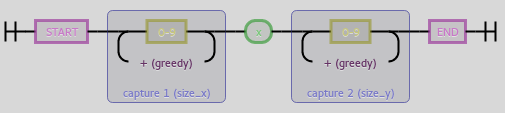^(?P<size_x>\d+)x(?P<size_y>\d+)$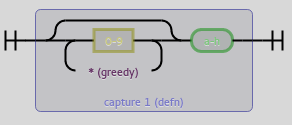(?P<defn>\d*[a-h])

Now we go on to parsing the problem. The parsing function should get the problem as a string and return a 3-tuple containing width and height of the problem as well as a mapping of positions within the grid to Node instances.

Onto actually parsing. We can split at the colon and then regular expressions to the rescue. The size definition has the form ^(?P<size_x>\d+)x(?P<size_y>\d+)$while a single node definition has the form (?P<defn>\d*[a-h]). As we can see, the digits are optional but we need to greedily accept them. All non-overlapping occurences in order will be our cell definitions. In code we, again, do nothing too too fancy: def parse(puzzle_str): directions = { 'a': (0,-1), 'b': (1,-1), 'c': (1,0), 'd': (1,1), 'e': (0,1), 'f': (-1,1), 'g': (-1,0),'h': (-1,-1) } size, _, definition = puzzle_str.partition(':') r_size = re.compile(r"^(?P<size_x>\d+)x(?P<size_y>\d+)$")
r_defn = re.compile(r"(?P<defn>\d*[a-h])")
size_t = tuple(map(int, r_size.match(size).groups()))
w, h = size_t
nodes = {}
for n, m in enumerate(r_defn.finditer(definition)):
pos = (n % w, n // w)
nodes[pos] = Node(directions[m.group(0)[-1:]])
hint = m.group(0)[:-1]
if hint:
nodes[pos].hint = int(hint)
return w, h, nodes


And we also need to fill in the successor Nodes. This is no problem at all.

w, h, nodes = parse(sys.argv)
from itertools import product
for x, y in product(range(w), range(h)):
this_node = nodes[(x,y)]
dx, dy = this_node.direction
x, y = x + dx, y + dy
while x >= 0 and x < w and y >= 0 and y < h:
this_node.following = this_node.following | frozenset([nodes[x,y]])
x, y = x + dx, y + dy


And now on to actually solving this thing. The idea is, that we slowly build a list of Nodes that is our solution. The first element is the Node with the hint "1" and we iterate through all nodes that follow that Node and do not have a hint that is other than "2", and so on. And of course, the Node that we add to the solution is not allowed to have already been part of the solution and if there is a node with that hint, it should be this exact one. If we find such a conflict in our solution, we reject that solution and return to an earlier partial solution.

Instead of using recursion, we build a queue of partial solutions until we get a complete solution. This is functionally identical to restarting a solver function with partial solutions of increasing size. In that case, the call stack manages the backtracking. But we'll do it with a queue this time. We build the queue so that every element in the list is either

1. a partial solution (beginning from 1) without conflicts
2. a partial solution (beginning from 1) where the last element is in some conflict
3. a complete solution

For case 1, we remove the partial solution that has the length n and add all partial solutions of length n+1 by iterating through all successor Nodes that have not yet been part of the partial solution. For case 2, we reject that partial solution. For case 3, we immediately return that solution and are finished.

def solve_dhc(nodes):
# get the Node that has hint 1
first = next(iter(n for n in nodes.values() if n.hint == 1))
# get the set of all hints, so it is easier to check for conflicts
hints = frozenset(n.hint for n in nodes.values() if n.hint is not None)
from collections import deque
q = deque([[first]]) # initializing queue
while q:
curr = q.pop()
idx = len(curr)
if (idx in hints or curr[-1].hint is not None) and curr[-1].hint != idx:
continue # case 2
if idx == len(nodes):
return curr # case 3
for _next in curr[-1].following: # case 1
if _next not in curr:
q.append(curr + [_next])


This algorithm terminates because we always remove one element from the queue of some length n and possibly add a large but finite amount of elements of length n+1 and the algorithm terminates when we have an element in the queue of some possibly large but finite length.

Sidenote: if you use popleft instead of pop you get a breadth-first search instead the depth-first search, that is implemented here. Add to the right and pop from the right for depth-first and add to the right and pop from the left for breadth-first. Since every proper solution has the exact same length/depth (that we know and never go further anyways), searching depth-first is strictly better than breadth-first.

And given a solution, we still have to print it. That's the easy part.

dhc = solve_dhc(nodes)

fill = len(str(w*h))
for l in range(h):
for c in range(w):
node = nodes[(c,l)]
print(str(dhc.index(node) + 1).rjust(fill), end=" ")
print("")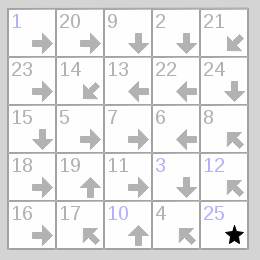Solved Puzzle

And there's the solution for our puzzle.

$python3 signpost_dhc.py 5x5:1cceefcfggeeccghcac3e12hch10ah25a 1 20 9 2 21 23 14 13 22 24 15 5 7 6 8 18 19 11 3 12 16 17 10 4 25  Find the code here: github.com/maweki/tatham-slv 3. Published: Sun 31 January 2016 # Solving Tatham's Puzzles - Pattern I've started writing solvers for games of Simon Tatham's Portable Puzzle Collection. Those Problems can usually be reduced to some NP-complete problem. This is quite a fun exercise. We will be starting with the puzzle "Pattern", also known as Nonograms that we reduce to SAT.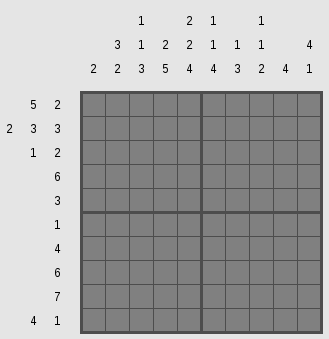Unsolved Nonogram First to explain the puzzle: The cells of the puzzle can be filled with black or white squares. The numbers on the sides of the rows and colums denote the number of consecutive black squares. Every run of black squares has to be seperated from another run by white squares. First we want to define a type for our problem. type PSize = (Int, Int) type Run = [[Int]] type Runs = (Run, Run) data Problem = Problem PSize Runs deriving Show  The puzzle on the side is defined in the Tatham format by the string 10x10:2/3.2/1.1.3/2.5/2.2.4/1.1.4/1.3/1.1.2/4/4.1/5.2/2.3.3/1.2/6/3/1/4/6/7/4.1. It has a size information and then the list of runs. Another format we want to support is the Haskell Runs format which would be ([,[3,2],[1,1,3],[2,5],[2,2,4],[1,1,4],[1,3],[1,1,2],,[4,1]],[[5,2],[2,3,3],[1,2],,,,,,,[4,1]]). This format seperates the runs for rows and columns and ommits the size information. For the second format we can use Haskell's own read function. For Tatham's format we have to define a parser. I've added type information for most functions which is often needed for code involving read. import Text.ParserCombinators.ReadP number' = many1 (choice (map char ['0'..'9'])) r_size :: ReadP PSize r_size = do l <- number' char 'x' r <- number' return (read l, read r) r_run :: ReadP [Int] r_run = do { li <- sepBy number' (char '.'); return$ map read li }

r_runs :: ReadP [[Int]]
r_runs = sepBy r_run (char '/')

r_problem :: ReadP Problem
r_problem = do size <- r_size; char ':'
runs <- r_runs
skipSpaces; eof
return $Problem size (take (fst size) runs, drop (fst size) runs)  What do we now do with such a problem? First we want to check it for consistency. If we got size information it should fit the runs and the numbers in the runs (and the spaces between them) shouldn't add up to more than the size. import qualified Prelude as P (all, (&&), not) consistent :: Problem -> Bool consistent (Problem size (xin, yin)) = consistent' size xin P.&& consistent' (swap size) yin consistent' (w, h) xs = (w == length xs) P.&& P.all (\x -> (sum x) + (length x - 1) <= h) xs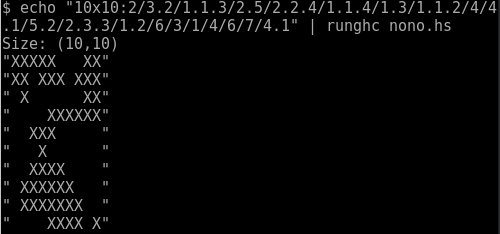Calling our solver But now on to solving the problem. We will reduce this problem to SAT using the Ersatz library. The encoding of a problem, I guess, is obvious. We say that every cell within the Nonogram is a Variable Bit and a bit being True has the semantics of that field being colored black while False would mean white. We want to build constraints of single rows or columns and then solve the conjunction of all those row/column-constraints for satisfiability. Therefore we define a function of the type [Int] -> [Bit] -> Bit that takes a list of Integers (the numbers of one column or row) and the list of remaining Bits that do not yet are constrained. First we begin with the end of the recursion. If no number is left or the remaining number is zero (for empty columns or rows), we want all remaining variables to be False. (we take for to be defined as flip map) build_runs :: [Int] -> [Bit] -> Bit build_runs nums vars = case nums of [] -> all not vars  -> all not vars x:xs -> let max_d = length vars - (length xs + sum xs) - x run = [not] ++ replicate x id ++ [not] v = [false] ++ vars ++ [false] in any id$ for [0..max_d] $\ d -> (all not$ take d vars)
&& (all id $zipWith id run$ take (x + 2) $drop d v) && (build_runs xs$ drop (x + d + 1) vars)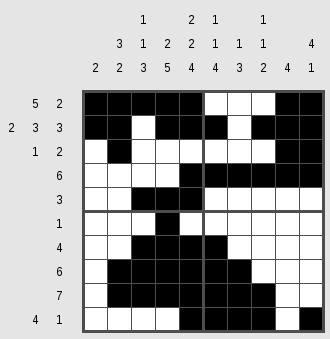Solved Nonogram

The recursion is something to think about a bit. We're given a number of consecutive black squares x and all the variables in the column/row vars. The x Trues can be put anywhere from 0 to max_d where max_d is all the rest of the variables take the minimum necessary space of the remaining number of numbers xs, while all variables before that number of black squares and one after need to be white. For every possible position the rest of the variables need to fulfill the same condition with the rest of the numbers.

Now we have to combine all the constraints from a given problem.

nono :: (MonadState s m, HasSAT s) => Problem -> m (Map (Int,Int) Bit)
nono (Problem (sizex, sizey) (xin, yin)) = do
let indices = [(x, y) | x <- [1..sizex], y <- [1..sizey] ]
vars' <- replicateM (length indices) exists
vars <- return $Map.fromList$ zip indices vars'
let getx x = for [1..sizey] $\y -> (vars ! (x,y)) gety y = for [1..sizex]$ \x -> (vars ! (x,y))
xs = zipWith build_runs xin (map getx [1..])
ys = zipWith build_runs yin (map gety [1..])
assert (all id xs && all id ys)
return vars


Internally we let Ersatz identify variables by their position in the grid Map (Int,Int) Bit. Now we can solve our problem easily using minisat with Ersatz's native minisat binding. Given a solution, we just format it correctly and give it to the user.

main = do
input <- getContents
let parsed = parse_tat input
problem = case parsed of
Just p -> p
Nothing -> let (xin, yin) = read input :: ([[Int]],[[Int]])
in Problem (length xin, length yin) (xin, yin)

putStrLn $"Size: " ++ (show$ problem_size problem)
unless (consistent problem) $do {putStrLn "Problem inconsitent"; exitFailure} (Satisfied, Just solution) <- solveWith minisat (nono problem) let sizey = snd$ problem_size problem
lines = for [1..sizey] $\i -> filter (\ ((x, y), b) -> y == i)$ toList solution
conv  = map \$ \((x,y), b)-> if b then 'X' else ' '
join = foldr (:) ""
forM_ (map (join . conv) lines) print


And we're done.

Find the code here: github.com/maweki/tatham-slv